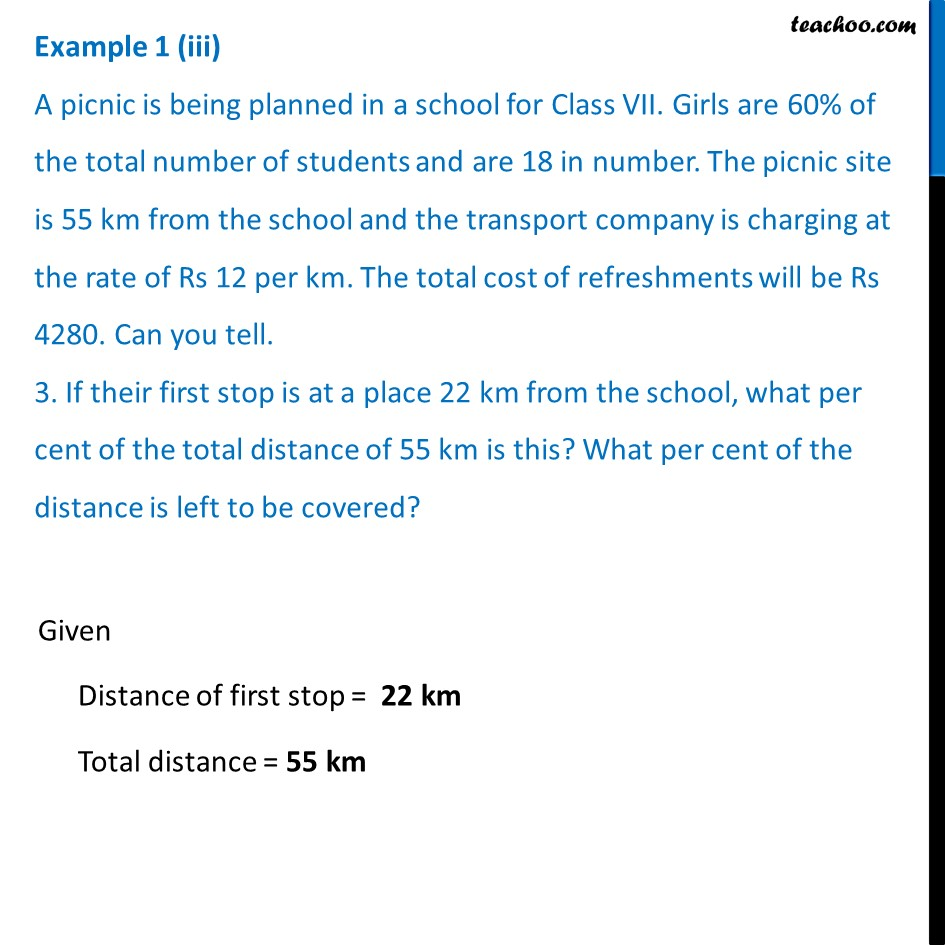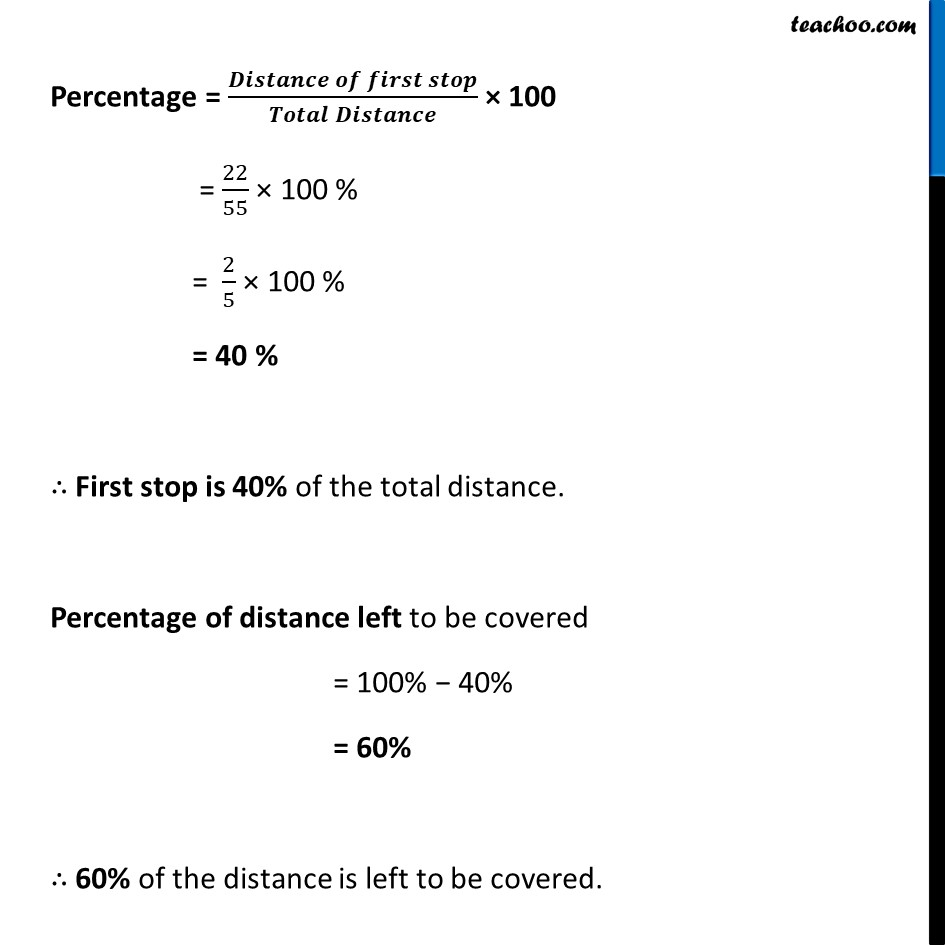Examples

Chapter 7 Class 8 Comparing Quantities
Serial order wiseLearn in your speed, with individual attention - Teachoo Maths 1-on-1 Class

### Transcript

Example 1 (iii) A picnic is being planned in a school for Class VII. Girls are 60% of the total number of students and are 18 in number. The picnic site is 55 km from the school and the transport company is charging at the rate of Rs 12 per km. The total cost of refreshments will be Rs 4280. Can you tell. 3. If their first stop is at a place 22 km from the school, what per cent of the total distance of 55 km is this? What per cent of the distance is left to be covered? Given Distance of first stop = 22 km Total distance = 55 km Percentage = (𝑫𝒊𝒔𝒕𝒂𝒏𝒄𝒆 𝒐𝒇 𝒇𝒊𝒓𝒔𝒕 𝒔𝒕𝒐𝒑)/(𝑻𝒐𝒕𝒂𝒍 𝑫𝒊𝒔𝒕𝒂𝒏𝒄𝒆) × 100 = 22/55 × 100 % = 2/5 × 100 % = 40 % ∴ First stop is 40% of the total distance. Percentage of distance left to be covered = 100% − 40% = 60% ∴ 60% of the distance is left to be covered.